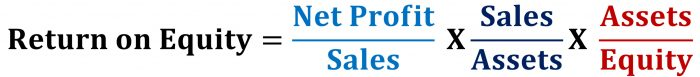## Is a Declining ROE always bad – The case of PI Industries

The ROE (Return on Equity) for PI Industries, one of India’s larger Chemical companies, fell from 17% in 2020 to 14% in 2021.

The profit had grown by 60% in 2021, while sales grew by 35%. So what happened to ROE?

Let’s decode this.

### What is ROE?

ROE = Net Profit / Equity

We can rewrite the equation as (by multiplying both numerator and denominator by the same factors)

ROE = (Net Profit / Equity) X (Sales / Sales) X (Assets / Assets)

Now, rearranging the terms, we can write it as

ROE = (Net Profit / Sales) X (Sales / Assets) X (Assets / Equity)The first term is Net Profit Margin
The second term is Asset Turnover Ratio
The third term is Equity Multiplier (Leverage Ratio)

The equation, as we may know, is called Dupont Analysis

Basically tells us about where the movement in ROE is coming from.

Now when you do this for PI Industries, you realize that NPM went up, but Asset Turnover and Equity Multiplier went down. And that was due to the firm raising a large amount of equity during the year. If you raise equity capital, this would increase equity, and cash, till the time you use it. The benefits of it would appear in the long run, but in the short run, it will depress the ROE. Not necessarily bad, right? A falling Return on Equity is not always bad.

Similarly , some companies may raise debt, thus increasing the leverage ratio, and the ROE would show a jump. Not necessarily good, since the impact may come in future years.

The simple Dupont analysis helps us decode ROE trends of companies. Try this for some other companies and see what happens?

### Recent Blog Posts

Delhivery listed recently on Indian stock exchanges. We wrote a note about Delhivery and India’s logistics market. Read it here